##### Calculus II For DummiesDifferential equations (DEs) come in many varieties. And different varieties of DEs can be solved using different methods. You can classify DEs as ordinary and partial Des. In addition to this distinction they can be further distinguished by their order.

Here are some examples: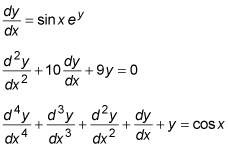Solving a differential equation means finding the value of the dependent variable in terms of the independent variable. The following examples use y as the dependent variable, so the goal in each problem is to solve for y in terms of x.

An ordinary differential equation (ODE) has only derivatives of one variable — that is, it has no partial derivatives. Here are a few examples of ODEs: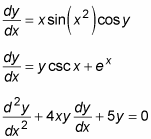In contrast, a partial differential equation (PDE) has at least one partial derivative. Here are a few examples of PDEs: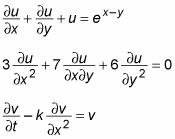DEs are further classified according to their order. This classification is similar to the classification of polynomial equations by degree.

First-order ODEs contain only first derivatives. For example: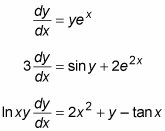Higher-order ODEs are classified, as polynomials are, by the greatest order of their derivatives. Here are examples of second-, third-, and fourth-order ODEs: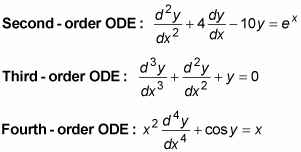As with polynomials, generally speaking, a higher-order DE is more difficult to solve than one of lower order.

What constitutes a linear differential equation depends slightly on who you ask. For practical purposes, a linear first-order DE fits into the following form: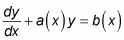where a(x) and b(x) are functions of x. Here are a few examples of linear first-order DEs: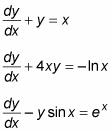Linear DEs can often be solved, or at least simplified, using an integrating factor.

A linear second-degree DE fits into the following form: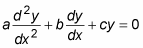where a, b, and c are all constants. Here are some examples: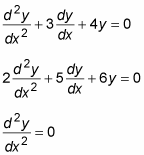Note that the constant a can always be reduced to 1, resulting in adjustments to the other two coefficients.